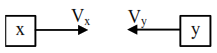# Two cars X and Y

Question:

Two cars $\mathrm{X}$ and $\mathrm{Y}$ are approaching each other with velocities $36 \mathrm{~km} / \mathrm{h}$ and $72 \mathrm{~km} / \mathrm{h}$ respectively. The frequency of a whistle sound as emitted by a passenger in car X, heard by the passenger in car $\mathrm{Y}$ is $1320 \mathrm{~Hz}$. If the velocity of sound in air is $340 \mathrm{~m} / \mathrm{s}$, the actual frequency of the whistle sound produced is ........ Hz.

Solution:$\mathrm{V}_{\mathrm{x}}=36 \mathrm{~km} / \mathrm{hr}=10 \mathrm{~m} / \mathrm{s}$

$\mathrm{V}_{\mathrm{y}}=72 \mathrm{~km} / \mathrm{hr}=20 \mathrm{~m} / \mathrm{s}$

by doppler's effect

$\mathrm{F}^{\prime}=\mathrm{F}_{0}\left(\frac{\mathrm{V} \pm \mathrm{V}_{0}}{\mathrm{~V} \pm \mathrm{V}_{\mathrm{s}}}\right)$

$1320=\mathrm{F}_{0}\left(\frac{340+20}{340-10}\right) \Rightarrow \mathrm{F}_{0}=1210 \mathrm{~Hz}$